# Easiest colleges to get into in Massachusetts

Top 10 colleges in Massachusetts with the highest acceptance rates
What are the easiest colleges to get into in Massachusetts? We've got you covered. We've compiled a national college database and have created a list of the easiest colleges to get into in Massachusetts below. These are the easiest 4 year colleges to get into in Massachusetts that have the highest acceptance rates, and are more likely to accept low GPAs, GEDs, and everything in between. There are tons of good colleges below that accept more applicants, and we also share their average SAT and ACT scores so that you can see where you're most competitive. Read on to find out more.

## Wheelock College acceptance rate

Wheelock College acceptance rate is 95%.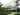The average SAT score for Wheelock College is 1030.

• SAT composite: 1030
• SAT math: 500

The average ACT score for Wheelock College is 20.

## Curry College acceptance rate

Curry College acceptance rate is 92.7%.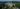The average SAT score for Curry College is 1026.

• SAT composite: 1026
• SAT math: 510

The average ACT score for Curry College is 21.

## Bridgewater State University acceptance rate

Bridgewater State University acceptance rate is 90.1%.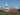The average SAT score for Bridgewater State University is 1070.

• SAT composite: 1070
• SAT math: 530

The average ACT score for Bridgewater State University is 22.

## Massachusetts Maritime Academy acceptance rate

Massachusetts Maritime Academy acceptance rate is 89.1%.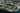The average SAT score for Massachusetts Maritime Academy is 1132.

• SAT composite: 1132
• SAT math: 570

The average ACT score for Massachusetts Maritime Academy is 23.

## Fitchburg State University acceptance rate

Fitchburg State University acceptance rate is 86.9%.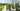The average SAT score for Fitchburg State University is 1072.

• SAT composite: 1072
• SAT math: 540

The average ACT score for Fitchburg State University is 21.

## Western New England University acceptance rate

Western New England University acceptance rate is 85.4%.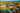The average SAT score for Western New England University is 1160.

• SAT composite: 1160
• SAT math: 580

The average ACT score for Western New England University is 24.

## Suffolk University acceptance rate

Suffolk University acceptance rate is 83.4%.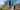The average SAT score for Suffolk University is 1086.

• SAT composite: 1086
• SAT math: 540

The average ACT score for Suffolk University is 24.

## Assumption College acceptance rate

Assumption College acceptance rate is 81.1%.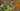The average SAT score for Assumption College is 1166.

• SAT composite: 1166
• SAT math: 580

The average ACT score for Assumption College is 26.

## Endicott College acceptance rate

Endicott College acceptance rate is 80.5%.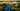The average SAT score for Endicott College is 1167.

• SAT composite: 1167
• SAT math: 580

The average ACT score for Endicott College is 24.

## Worcester State University acceptance rate

Worcester State University acceptance rate is 77.8%.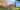The average SAT score for Worcester State University is 1102.

• SAT composite: 1102
• SAT math: 550